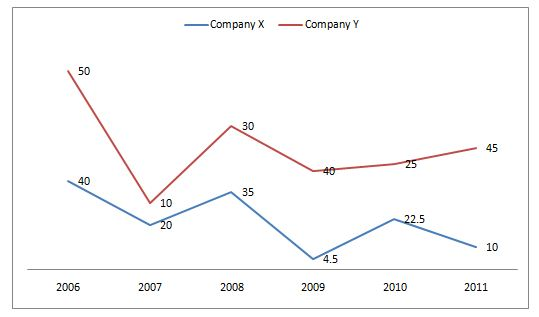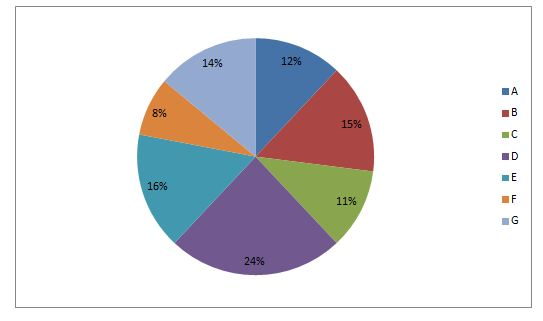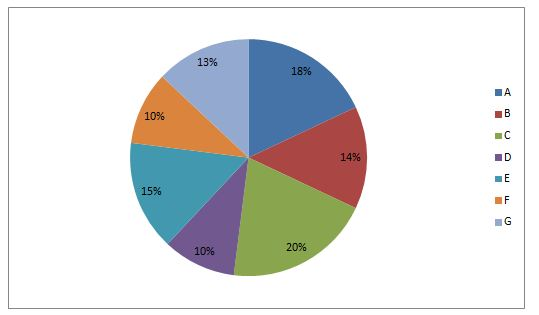# Crack SBI Clerk Prelims 2018 Sectional Full Test (Quantitative Aptitude) Day-2

Dear Readers, SBI Clerk (Junior Associates) 2018 Preliminary Examination is scheduled to be held on 23rd, 24th, and 30th of June 2018. In that case, aspirants need to speed up the preparation as there are only few days more.

To boost up your exam preparation, Our IBPS Guide team is providing a full length Sectional Tests for English, Quantitative Aptitude and Reasoning with detailed solutions to score more marks in the prelims exam. Make use of this opportunity and recommend to your friends to achieve a successful career in Banking.

You can also practice using our SBI Clerk Test Series pack prepared by our experienced professionals as per the latest exam pattern. We wish you a great success in all forthcoming exams. Click Here for SBI Clerk Practice Mock Test

#### Minimum Cut Off for this Quantitative Aptitude Section Test is: 20

[WpProQuiz 2474]

Click “Start Quiz” to attend these Questions and view Solutions

Directions (1-5): Study the following and answer the following questions.

The following line graph shows that the profit percent of two companies X and Y in 2006 to 2011Note: profit percentage= [(income-expenditure)/expenditure]*100

1. If the incomes of the two companies X and Y in 2007 were equal, then what was the ratio of the expenditure of the companies X and Y in 2007?
1. 13:12
2. 13:15
3. 15:12
4. 11:12
5. 14:17
1. The income of company Y in 2006 was Rs 63 lakhs. If the expenditure of company Y in 2008 was half the expenditure of company Y in 2006, then what was the income of company Y in 2008?
1. Rs 72.3 lakhs
2. Rs 62.3 lakhs
3. Rs 57.3 lakhs
4. Rs 87.3 lakhs
5. Rs 27.3 lakhs
1. If the profit of company X in 2010 is Rs 67.5 lakhs, then what is the profit of the same company in 2011, whose expenditure is 2/3rd of the expenditure of company X in 2010?
1. Rs 20 lakhs
2. Rs 18 lakhs
3. Rs 15 lakhs
4. Rs 23 lakhs
5. Rs 31 lakhs
1. Total income of company Y in all the given years together was Rs 2340 lakhs. What was the total expenditure of company Y in all the given years together?
1. Rs 52 lakhs
2. Rs 41 lakhs
3. Rs 63 lakhs
4. Rs 45 lakhs
5. Cannot be determined
1. If the incomes of Company X in 2008 and 2011 were in the ratio 5:7, then what was the ratio of expenditure of the company in these two years?
1. 110/185
2. 114/189
3. 113/184
4. 111/186
5. 110/189
1. The ratio of the ages of Manu and Salim 15 years ago was 2:5. The ratio of their ages 5 years hence will be 3:5. Find the ratio of their present ages.
1. 12:41
2. 23:31
3. 62:21
4. 21:98
5. 31:55
1. What is the cost of covering a spherical bowl of radius 35 meters with a golden sheet cover at the rate of Rs 1.5/m2.
1. Rs 23300
2. Rs 13400
3. Rs 32700
4. Rs 23100
5. Rs 12700
1. The ratio of prices of Two TV’s LG and Samsung in the last year was 4:3. In this year, the price of LG is decreased by Rs 10000 and that of Samsung increased by 20 percent, their prices are now in the ratio 5:6, then find the price of LG TV in the last year.
1. Rs 20000
2. Rs 92000
3. Rs 40000
4. Rs 26000
5. Rs 50000
1. A man can row 28 km/hr in still water. It takes him thrice as long to move upstream as to downstream the river. Then find the rate of stream.
1. 32 km/hr
2. 34 km/hr
3. 14 km/hr
4. 17 km/hr
5. 21 km/hr
1. On selling 400 pens at Rs 3825, there is a profit equal to the cost price of 50 pens. What is the cost price of a pen?
1. Rs 8.5
2. Rs 6
3. Rs 10
4. Rs 7.5
5. Rs 11
1. If the sum of the ages of Nancy and Sofi and twice the age of Mithun, the total age becomes 89. If the sum of the ages of Mithun and Sofi and thrice the age of Nancy, the total age becomes 98 and if the age of Nancy is added to thrice the age of Mithun and thrice the age of Sofi, the total age becomes 154. What is the age of Nancy?
1. 20 years
2. 27 years
3. 21 years
4. 15 years
5. None of these
1. Abi earns an interest Rs 5124 for the third year and Rs 4270 for the second year on the sum of Rs.4720. Find the rate of interest if it is lent at simple interest.
1. 15%
2. 40%
3. 50%
4. 20%
5. 10%
1. In covering a distance of 180 km, Fathima takes 4 hours more than Vinay. If Fathima doubles her speed, then she would take 2 hour less than Vinay. What is the speed of Fathima?
1. 13 km/hr
2. 19 km/hr
3. 11 km/hr
4. 15km/hr
5. 14 km/hr
1. Two numbers in the form x/y is in such a way that y is 20% more than x and product of them is 2430. Find the sum of x and y.
1. 99
2. 93
3. 86
4. 69
5. 93
1. A train can cross a platform of 270 m length completely in 18 seconds while it covers a platform of double of its length in 27 seconds. Find the speed of the train.
1. 62 m/s
2. 25 m/s
3. 30 m/s
4. 14 m/s
5. 53 m/s

Directions (16-20): What will come in place of question mark in the following questions?

1. (41.99% of 6100.039+83.83) ÷ (30.039% of 1349.847-5.993) =?
1. 52
2. 27
3. 14
4. 16
5. 8
1. (8262.0142/53.784)+ (624.0203/12.37)*(7156.933/421.028) = 33.99*?
1. 31
2. 14
3. 16
4. 20
5. 60
1. (4175.98)*(3.01/4.32)*(5.02/5.89)*7.09 =? +(16*13)
1. 18335
2. 18035
3. 18284
4. 18062
5. 18328
1. 18.982*23.010-197.01+152.90=?
1. 273
2. 187
3. 726
4. 632
5. 393
1. (699.9/269.99)*404.99+575.9/12.01=?
1. 1683
2. 1098
3. 1769
4. 1573
5. 1637

Directions (21-25): In each of the following questions, two equations (i) and (ii) are given. You have to solve them and find the correct option.

1. x>y
2. x<y
3. x ≤ y
4. x ≥y
5. x=y Or cannot be determined

21 a) 45x+24y-56=0

b) 10x+ 6y-13=0

22.a)21x= 517+470

b)y= (√(6181-6132))2

23.a)x2= 527+351+643

b) y2+15y-324=0

24).

a)5x2+11x+6=0

b) 6y2+11y+5=0

25).

a) x= √(192-37)

b) y=(√484+√1024)/(√3)2

Directions (26-30): Study the following and answer the following questions.

The following pie charts Show the distribution of students in Primary and Secondary levels in seven different schools.Total number of students in Primary level= 32700Total number of students studying in Secondary level= 42600

26.The number of students studying in the Primary level is approximately what percentage more than that of Secondary level from School D?

1. 43%
2. 47%
3. 73%
4. 84%
5. 53%
1. What is the total number of students studying at Secondary level from schools A, C and G?
1. 21235
2. 21462
3. 22566
4. 24626
5. 21726
1. Total number of students studying primary level in school F is approximately what percent of total number of students studying primary level of School G?
1. 58%
2. 35%
3. 57%
4. 62%
5. 26%
1. What is the ratio between the number of students studying Secondary level from School C and the number of students studying at Primary level from School E?
1. 323:211
2. 324:211
3. 263:219
4. 355:218
5. 373:215
1. If half of the total number of students studying in secondary level in Schools A, B, C, D and E has to attend the Camp, then find the number of students in Secondary level is not attending the camp?
1. 16401
2. 16241
3. 16421
4. 19271
5. 19321

Directions (31-35): Find the missing number in the following number series?

1. 15.6, 16.3, 17.7, 19.8, 22.6, ?
1. 26.1
2. 28.3
3. 25.4
4. 27.7
5. None of these

32. 750,1125,1500,1875, 2250,?

1. 2555
2. 2975
3. 2625
4. 2875
5. None of these
1. 2, 8, 17, 30.5, 50.75, ?
1. 124.5
2. 125
3. 125.75
4. 81.125
5. None of these
1. 9, 5, 9, 25, 91, ?
1. 432
2. 414
3. 420
4. 415
5. None of these
1. 21, 25, 34, 59, 108, ?
1. 218
2. 220
3. 225
4. 229
5. None of these

Let the income of the companies X and Y in 2007 be Rs x.

Also Let us take the expenditure of the company X be E1 and that of the company Y be E2.

Now, 20= [(x- E1)/ E1]*100

x=120 E1/100………….. (1)

Also for company Y,

10 = [(x- E2)/ E2]*100

x= 110 E2/100………….. (2)

From (1) and (2), we have

120 E1/100=110 E2/100

E1/ E2= 110/120

=11/12

Hence E1: E2= 11:12

Given that the income of company Y in 2006= Rs 63 lakhs and profit percentage= 50%

Let Rs x be the expenditure of the company Y in 2006.

Then, 50= [(63-x)/x]*100

x = (63-x)*2

3x= 126

x=Rs 42 lakhs

Then in 2008, the expenditure of the company Y= Rs 21 lakhs

Let y be the income of the company Y in 2008.

Then, 30 = [(y-21)/21]*100

63= 10y-210

y= Rs 27.3 lakhs

Let x be the expenditure of the company X in 2010.

Given that, the profit of company X in 2010= Rs 67.5 lakhs

(i.e) 22.5/100*x=67.5

x= Rs 300 lakhs

Then the expenditure of the company X in 2011= Rs 200 crores

Now, profit of the company X in 2011= 200*10/100

= Rs 20 lakhs

Since we cannot find the income of a company Y for all the years separately, the total expenditure of the same company will not be determined.

Given that, Income2008/ Income2011= 5/7

Since the profit percentage of a company X in 2008 is 35% and that of in 2011 is 10%, we have

135% of Expenditure2008/110 % of Expenditure 2011= 5/7

(i.e) Expenditure2008/ Expenditure 2011= 5/7*110/135

= 110/189

Let the ages of Manu and Salim 15 years ago be 2x and 5x respectively.

Then, the present age of Manu= (2x+15)

And the present age of Salim= (5x+15)

Now, (2x+15+5)/ (5x+15+5) =3/5

(2x+20)/(5x+20)=3/5

5(2x+20) =3(5x+20)

x=8

Thus present age of Manu= (2x+15) =31

Present age of Salim= (5x+15) =55

Required ratio= 31:55

We know that surface area of the sphere= 4πr2.

= 4*22/7*35*35

=15400 m2

Then the cost of covering the spherical bowl= (15400*1.5) = Rs 23100

Let the price of LG TV in the last year be 4x and the price of Samsung TV in the last year be 3x.

Then, (4x-10000)/(3x*120/100)=5/6

6(4x-10000) =5(3x*6/5)

6x= 60000

x= 10000

Thus the price of LG TV in the last year =4x= Rs 40000

Let the rate of upstream be x km/hr and then rate of downstream be 3x km/hr.

Then, 2x= 28

x= 14 km/hr

(i.e) Rate of upstream= 14 km/hr

And rate of downstream= 42 km/hr

Thus, Rate of stream= ½(42-14)=1/2 *(28)= 14 km/hr

Given that profit= Cost price of 50 pens

Now, Total profit= Selling price of 400 pens- Cost price of 400 pens

Cost price of 50 pens = 3825 – Cost price of 400 pens

Cost price of 50 pens+ Cost price of 400 pens=3825

Cost price of 450 pen =3825

Thus, Cost price of 1 pen= 3825/450= Rs 8.5

Let x be age of Nancy, y be the age of Sofi and z be the age of Mithun.

According to the question, x+y+2z=89 ……………. (1)

3x+y+z= 98 …………… (2)

x+3y+3z=154 …………. (3)

After solving the equations, we have x= 35/2 = 17.5 years.

Let r be the rate of interest.

For question, we have simple interest for 1 year= 5124-4270=Rs 854

Then, 854= (4270*r)/100

r= 854*100/4270

r= 20%

Let the speed of Fathima be x km/hr

Then, 180/x-180/2x =6

(360-180)/2x =6

180=12x

x=15km/hr

Given that y= 120x/100 ….. (1)

Also xy=2430 … (2)

Substituting (1) in (2), we have

120x2/100=2430

x2= 2430*100/120

=2025

x=45

Then, y= 54

Thus, x+y =99

Let x be the length of the train

(x+270)/18=3x/27=speed

(x+270)/18= x/9

x+270=2x

x= 270

Thus speed of the train=3*270/27 = 30 m/s

Directions (16-20):

(42% of 6100+84)÷ (30% of 1350-63) =?

(2562+84) ÷ (405-216) =?

?=14

153+ (52*17) =34 *?

153 + 884= 34* ?

1037/34=?

?= 31

4176*3/4*5/6*7=?+ 208

18270-208=?

18062=?

19*23-197+153=?

437-197+153=?

393=?

700/270*405+576/12=?

1050+48=?

1098=?

Directions (21-25):

45x+24y-56=0 …………… (1)

10x+ 6y-13=0 …………….. (2)

Multiplying equation (2) by 4, then we have

40x+24y-52=0 ………….. (3)

Solving (1) and (3), we have x= 4/5 and y= 5/6

Hence x<y

a)21x= 517+470

21x= 987

x=47

b)y= (√(6181-6132))2

y= (√(49))2

y= 49

Hence x<y

a)x2= 527+351+643

x2=1521

x=39 and -39

b) y2+15y-324=0

y2+27y-12y-324=0

(y+27)(y – 12)=0

y= -27 and 12

Hence the relationship cannot be determined

a) 5x2+11x+6=0

5x2+5x+6x+6=0

(x+1)(5x+6)=0

x= -1 and -6/5

b) 6y2+11y+5=0

6y2+6y+5y+5=0

(6y+5)(y+1)=0

y= -1 and -5/6

Hence x≤y

a) x= √(192-37)

x= √(361-37)

x=√324

x= 18

b) y=(√484+√1024)/(√3)2

y= (√484+√1024)/(√3)2

y= (22+32)/3

y=54/3

y= 18

Hence x=y

Directions (26-30):

Number of students studying in the Secondary level in school D=10/100*42600

= 4260

Similarly, number of students studying in the Primary level in school D=24/100*32700

= 7848

Required percentage=3588/4260*100

= 84%

Number of students studying in the Secondary level in school A=18/100*42600

= 7668

Number of students studying in the Secondary level in school C=20/100*42600

= 8520

Number of students studying in the Secondary level in school G=13/100*42600

= 5538

Total number of students studying at Secondary level from A, C and G=7668+8520+5538=21726

Number of students studying in the Primary level in school F=8/100*32700

= 2616

Number of students studying in the Primary level in school G=14/100*32700

= 4578

Required percentage= 2616/4578*100=57%

Number of students studying in the Secondary level in school C=20/100*42600

= 8520

Total number of students studying primary level from school E=16/100*32700

= 5232

Required ratio= 8520:5232

= 355:218

Number of students studying in the Secondary level in school A=18/100*42600

= 7668

Number of students studying in the Secondary level in school B=14/100*42600

= 5964

Number of students studying in the Secondary level in school C=20/100*42600

= 8520

Number of students studying in the Secondary level in school D=10/100*42600

= 4260

Number of students studying in the Secondary level in school E=15/100*42600

= 6390

Total number of students studying secondary level from those schools= 7668+5964+8520+4260+6390                                                                                                                              = 32802

Thus Number of students not attending the camp= 32802/2=16401

Directions (31-35):

15.6+0.7 =16.3

16.3+1.4 =17.7

17.7+2.1=19.8

19.8+2.8= 22.6

22.6+3.5= 26.1

750/2 *3 =1125

1125/3 *4 = 1500

1500/4*5 = 1875

1875/5*6 = 2250

2250/6*7 =2625

(or)

The difference of the difference is 375,

Series follows,

*1.5 + 5